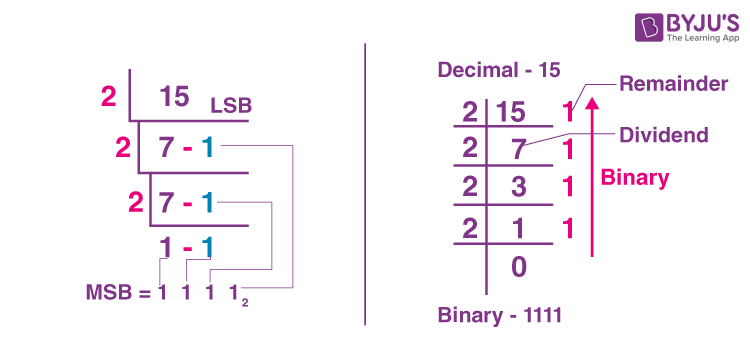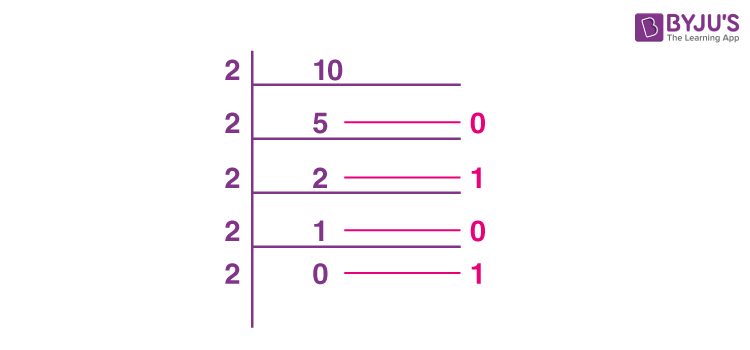# 15 in Binary

Binary and decimal numbers are the most commonly used number systems in mathematics. Let’s have a brief introduction of both binary and decimal number systems here. Binary numbers have base 2. Binary numbers are generally used in computer applications, where only two symbols or digits represent binary numbers, i.e. 0 (zero) and 1 (one). The binary numbers here are expressed in the base-2 numeral system. For example, (20)2 is a binary number. Each digit in this system is said to be a bit.

In the decimal number system, the numbers are represented with base 10. The way of denoting the decimal numbers with base 10 is also termed as decimal notation. Hence, it is also called the base-10 number system, consisting of 10 digits, such as 0, 1, 2, 3, 4, 5, 6, 7, 8, 9. This number system is widely used in computer applications. Each digit in the decimal system has a position, and every digit is ten times more significant than the previous digit.

However, it is possible to convert numbers from decimal to binary and binary to decimal. In this article, you will learn how to convert the decimal number 15 to a binary system and the binary equivalent of 15.

## 15 in Binary Number System

The binary equivalent of 15 is 1111. That means, the decimal number 15 can be written in the binary system as 1111. Since, we know that the binary number system contains the representation of numbers in combination with 0’s and 1’s. Based on the counting in the binary system, we can express 15 as 1111 in binary code.

## How to convert 15 to Binary

The binary equivalent of 15 is 1111. As we know, to convert any number from the decimal system to binary, we have to divide the number by 2 and keep track of the remainder. To convert decimal to binary numbers, proceed with the steps given below:

• Divide the given decimal number by “2”, where it provides the result along with the remainder.
• If the given decimal number is even, then the result will be a whole number, and it provides the remainder with “0.”
• If the given decimal number is odd, then the result is not appropriately divided, and it provides the remainder with “1”.
• By placing all the remainders in order in such a way, the Least Significant Bit (LSB) at the top and Most Significant Bit (MSB) at the bottom, the required binary number will be obtained.

The below figure shows the conversion of the number 15 to binary number as per the steps given above.### 0 to 15 in Binary

Let’s have a look at the table given below to know the conversion of decimal numbers from 0 to 15 in the Binary system.

 Decimal Binary 0 0 1 1 2 10 3 11 4 100 5 101 6 110 7 111 8 1000 9 1001 10 1010 11 1011 12 1100 13 1101 14 1110 15 1111

### What is 10 in Binary?

We can represent the decimal number 10 in the binary system as 1010, i.e. (10)10 = (1010)2.

This can be shown using the division as:Therefore, (10)10 = (1010)2

### How to count in Binary

Counting in the binary system starts with 0 and then goes to 1. Then we should add another digit as we do in decimal counting when we go from 9 to 10. Finally, we have to add another digit, so we have two digits now. So, in binary, we go from 1 to 10 since 1 is our last counting number. We go from 9 to 10 since 9 is the last number we can use when counting decimal numbers. So, every time we reach the last counting number, we have to add another digit to the previous number.

This can be done as follows:

0 → 0

1 → 1

10 → 2

11 → 3

100 → 4

101 → 5

110 → 6

111 → 7

1000 → 8

1001 → 9

1010 → 10

1011 → 12

Here, the left side of arrows represents binary numbers, and the corresponding decimal numbers are on the right side.

## Frequently Asked Questions – FAQs

Q1

### How would you express 15 in binary?

In the binary number system, 15 can be expressed as (1111)_2. Here, 2 is the base.

Q2

### What is the binary number of decimal number 15?

The binary number equivalent to the decimal number 15 is 1111.

Q3

### What does 10101 mean in binary?

The binary number 10101 means 21 in the decimal system.

Q4

### What does 11111 mean in binary?

The binary number 11111 means 31 in the decimal system.

Q5

### How do you write 1 in binary?

We can write 1 in binary as 1 or 0001.